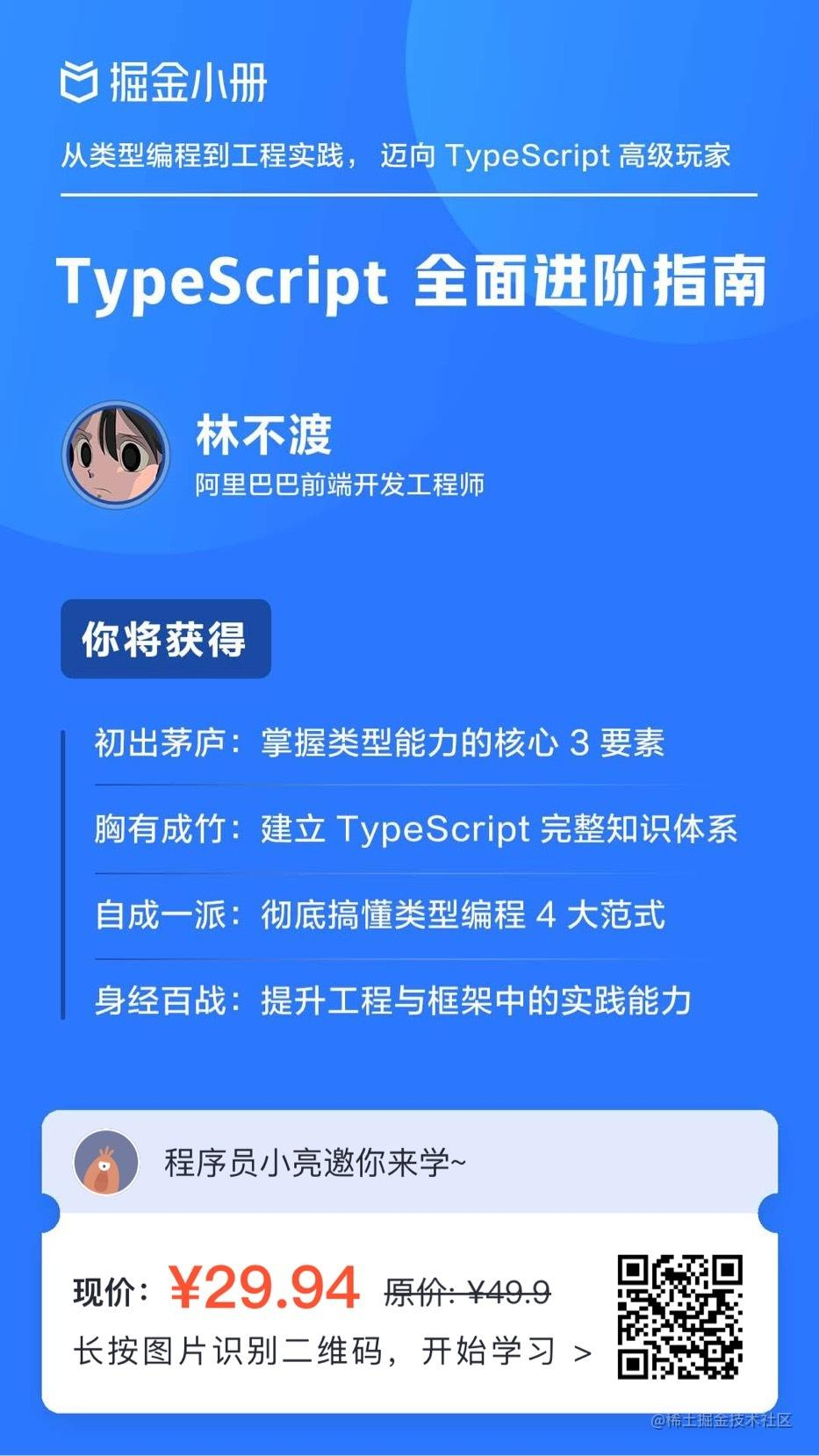#### 资源

```import 'dart:math' show Random;

main() async {
print('Compute π using the Monte Carlo method.');
await for (var estimate in computePi().take(500)) {
print('π ≅ \$estimate');
}
}

/// Generates a stream of increasingly accurate estimates of π.
Stream<double> computePi({int batch = 100000}) async* {
var total = 0;
var count = 0;
while (true) {
var points = generateRandom().take(batch);
var inside = points.where((p) => p.isInsideUnitCircle);
total += batch;
count += inside.length;
var ratio = count / total;
// Area of a circle is A = π⋅r², therefore π = A/r².
// So, when given random points with x ∈ <0,1>,
// y ∈ <0,1>, the ratio of those inside a unit circle
// should approach π / 4. Therefore, the value of π
// should be:
yield ratio * 4;
}
}

Iterable<Point> generateRandom([int seed]) sync* {
final random = Random(seed);
while (true) {
yield Point(random.nextDouble(), random.nextDouble());
}
}

class Point {
final double x, y;
const Point(this.x, this.y);
bool get isInsideUnitCircle => x * x + y * y <= 1;
}```

# 为什么使用 Dart ?

Google及全球的其他开发者，使用 Dart 开发了一系列高质量、 关键的 iOS、Android 和 web 应用。 Dart 非常适合移动和 web 应用的开发。

Dart 是免费的并开源于 GitHub。## 高效

Dart 语法清晰简洁，工具简单而强大。 输入检测可帮助您尽早识别细微错误。 Dart 拥有久经考验的 核心库（core libraries） 和一个已经拥有数以千计的 packages 生态系统

## 快速

Dart 提供提前编译优化，以在移动设备和 web 上实现可预测的高性能和快速启动。

## 可移植

Dart 可以编译成 ARM 和 x86 代码，因此 Dart 移动应用程序可以在 iOS，Android 及 更高版本上实现本地运行。 对于 web 应用程序，Dart 可以转换为 JavaScript。

## 易学

Dart 是面向对象的编程语言，语法风格对于许多现有的开发人员来说都很熟悉。 如果您已经了解 C++，C＃ 或 Java ，那么使用 Dart 也就是分分钟的事情。

## 响应式

Dart 可以便捷的进行响应式编程。由于快速对象分配和垃圾收集器的实现， 对于管理短期对象（比如 UI 小部件）， Dart 更加高效。 Dart 可以通过 Future Stream 的特性和API实现异步编程。